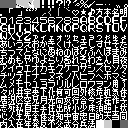News: 11 March 2016 - Forum Rules
Current Moderators - DarkSol, KingMike, MathOnNapkins, Azkadellia, Danke

###Author Topic: Kanji identification  (Read 3558 times)

#### FCandChill

• Hero Member
•• Posts: 555##### Kanji identification
« on: September 23, 2018, 02:59:44 pm »
Hi all. I'm making an script editor for a game. Here's the bank with all the characters...And here's the table file I have to far...

Code: [Select]
`00=[BLACK 1]01=[BLACK 2]02=[???1]03=[???2]04=[???3]05=！06=[！！]07=？08=[！？]09=[TILE 09]0A=％0B=／0C=―0D=‥0E=゛0F=゜10=．11=「12=」13=“14=”15=[WINDOW 15]16=[WINDOW 16]17=[WINDOW 17]18=[WINDOW 18]19=[Heart]1A=★1B=♪1C=[KANJI 1C]1D=[KANJI 1D]1E=[KANJI 1E]1F=[KANJI 1F]20=０21=１22=２23=３24=４25=５26=６27=７28=８29=９2A=Ａ2B=Ｂ2C=Ｃ2D=Ｄ2E=Ｅ2F=Ｆ30=Ｇ31=Ｈ32=Ｉ33=Ｊ34=Ｋ35=Ｌ36=Ｍ37=Ｎ38=Ｏ39=Ｐ3A=Ｑ3B=Ｒ3C=Ｓ3D=Ｔ3E=Ｕ3F=Ｖ40=Ｗ41=Ｘ42=Ｙ43=Ｚ44=ァ45=ィ46=ェ47=ォ48=ッ49=ャ4A=ュ4B=ョ4C=っ4D=ゃ4E=ゅ4F=ょ50=あ51=い52=う53=え54=お55=か56=き57=く58=け59=こ5A=さ5B=し5C=す5D=せ5E=そ5F=た60=ち61=つ62=て63=と64=な65=に66=ぬ67=ね68=の69=は6A=ひ6B=ふ6C=へ6D=ほ6E=ま6F=み70=む71=め72=も73=や74=ゆ75=よ76=ら77=り78=る79=れ7A=ろ7B=わ7C=を7D=ん7E=[KANJI 7E]7F=[KANJI 7F]80=ア81=イ82=ウ83=エ84=オ85=カ86=キ87=ク88=ケ89=コ8A=サ8B=シ8C=ス8D=セ8E=ソ8F=タ90=チ91=ツ92=テ93=ト94=ナ95=ニ96=ヌ97=ネ98=ノ99=ハ9A=ヒ9B=フ9C=ヘ9D=ホ9E=マ9F=ミA0=ムA1=メA2=モA3=ヤA4=ユA5=ヨA6=ラA7=リA8=ルA9=レAA=ロAB=ワAC=ヲAD=ンAE=[KANJI AE]AF=[KANJI AF]B0=々B1=[KANJI B1]B2=[KANJI B2]B3=[KANJI B3]B4=[KANJI B4]B5=[KANJI B5]B6=[KANJI B6]B7=[KANJI B7]B8=[KANJI B8]B9=[KANJI B9]BA=[KANJI BA]BB=[KANJI BB]BC=[KANJI BC]BD=[KANJI BD]BE=[KANJI BE]BF=[KANJI BF]C0=[KANJI C0]C1=[KANJI C1]C2=[KANJI C2]C3=[KANJI C3]C4=[KANJI C4]C5=[KANJI C5]C6=[KANJI C6]C7=[KANJI C7]C8=[KANJI C8]C9=[KANJI C9]CA=[KANJI CA]CB=[KANJI CB]CC=[KANJI CC]CD=[KANJI CD]CE=[KANJI CE]CF=[KANJI CF]D0=[KANJI D0]D1=[KANJI D1]D2=[KANJI D2]D3=[KANJI D3]D4=[KANJI D4]D5=[KANJI D5]D6=[KANJI D6]D7=[KANJI D7]D8=[KANJI D8]D9=[KANJI D9]DA=[KANJI DA]DB=[KANJI DB]DC=[KANJI DC]DD=[KANJI DD]DE=[KANJI DE]DF=[KANJI DF]E0=[KANJI E0]E1=[KANJI E1]E2=[KANJI E2]E3=[KANJI E3]E4=[KANJI E4]E5=[KANJI E5]E6=[KANJI E6]E7=[KANJI E7]E8=[KANJI E8]E9=[KANJI E9]EA=[KANJI EA]EB=[KANJI EB]EC=[KANJI EC]ED=[KANJI ED]EE=[KANJI EE]EF=[KANJI EF]F0=[KANJI F0]F1=[KANJI F1]F2=[KANJI F2]F3=[KANJI F3]F4=[KANJI F4]F5=[KANJI F5]F6=[KANJI F6]F7=[KANJI F7]F8=[KANJI F8]F9=[KANJI F9]FA=[KANJI FA]FB=[KANJI FB]FC=FD=FE=　FF=[BLACK 3]`
« Last Edit: September 25, 2018, 06:16:56 pm by FCandChill »

#### tashi

• Jr. Member
•• Posts: 66##### Re: Kaji identification
« Reply #1 on: September 23, 2018, 09:40:11 pm »
I'm afraid I'm at a loss for AF=[KANJI AF]
Maybe if you see it in context or in-game or something I might recognize it.

I wonder if a different tile editor would make the characters more readable?
The main block is in alphabetical order so it was pretty easy to guess the characters, but it would've been difficult if they were unsorted.

This is the main block:
々以井宇英下化何可回外核危机気休

And the interspersed ones:
Code: [Select]
`1C=[KANJI 1C] 方1D=[KANJI 1D] 本1E=[KANJI 1E] 名1F=[KANJI 1F] 明...7E=[KANJI 7E] 目7F=[KANJI 7F] 夜...AE=[KANJI AE] 用`

#### FCandChill

• Hero Member
•• Posts: 555##### Re: Kanji identification
« Reply #2 on: September 25, 2018, 07:36:22 am »
I'm afraid I'm at a loss for AF=[KANJI AF]
Maybe if you see it in context or in-game or something I might recognize it.

Thanks for the help! Here's one of the lines that uses the [KANJI AF]...

Code: [Select]
`―　メタルスレイタ゛―　全き発かんしゅうふつう[KANJI AF]　―`
« Last Edit: September 25, 2018, 06:17:05 pm by FCandChill »

#### tashi

• Jr. Member
•• Posts: 66##### Re: Kanji identification
« Reply #3 on: September 25, 2018, 07:31:49 pm »
It's probably 了 ... doesn't exactly make a lot of sense that I can tell. But it's probably right.

#### FCandChill

• Hero Member
•• Posts: 555##### Re: Kanji identification
« Reply #4 on: September 25, 2018, 07:41:44 pm »
Thanks, but it seems like you're unsure. Here's all the lines that use this character...

Code: [Select]
`―　メタルスレイタ゛―　全き発かんしゅうふつう[KANJI AF]　―また　　―　ふたい　てきと　せっしょくらないしますひとつ　―また　`
Code: [Select]
`[KANJI AF]かい！‥‥忠さん回ひを　かす゛おおくするとし゛かんをくいますてきか゛きたらわ　こちらからうってか゛　入っ出ましょう゛か`
Code: [Select]
`とウ゛ィウ゛ァ―チェは[KANJI AF]かい！と‥‥と何か゛　はし゛まるんた゛ろ‥‥やっ[TILE 09]し゛ゃ　ないて゛た゛め　　きかない‥‥とつきゃっ　くっ　くる！！`
Code: [Select]
`‥‥[KANJI AF]かい‥‥`
Code: [Select]
`[KANJI AF]かい！`
Code: [Select]
`[KANJI AF]かい！‥‥て゛も‥‥あっ　き　きます！`

#### tashi

• Jr. Member
•• Posts: 66##### Re: Kanji identification
« Reply #5 on: September 25, 2018, 10:32:19 pm »
Yeah, it's 100% 了
The font made it kinda hard to tell. I would've expected it to look like the 子 a few lines below it, just without the middle horizontal line, but the tiny font tile makes it look completely different, shifted to the right and shorter on top.

The tone of the first example line you gave made me think military-ish, so they'd probably say 終了 and 了解 and stuff, but I don't understand what they're actually saying, so I couldn't be sure from that alone.

Every additional sample you shared is 了解　了かい or "roger" so I'm confident it's correct now.

#### FCandChill

• Hero Member
•• Posts: 555##### Re: Kanji identification
« Reply #6 on: September 26, 2018, 09:42:41 pm »
Thanks for the help, tashi!